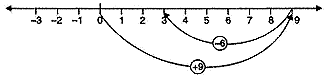# Extra Questions for Class 6 Math Integers Chapter 6

Extra Questions for Class 6 Math Integers Chapter 6. myCBSEguide has just released Chapter Wise Question Answers for class 6 Maths. There chapter wise Practice Questions with complete solutions are available for download in myCBSEguide website and mobile app. These Extra Questions with solution are prepared by our team of expert teachers who are teaching grade in CBSE schools for years. There are around 4-5 set of solved Mathematics Extra questions from each and every chapter. The students will not miss any concept in these Chapter wise question that are specially designed to tackle Exam. We have taken care of every single concept given in CBSE Class 6 Mathematics syllabus and questions are framed as per the latest marking scheme and blue print issued by CBSE for Class 6.

CBSE Class 6 Maths Extra Questions

## Important Questions of Class 6 Chapter 6 Mathematics

Ch-6 Integers

1. Write numbers with appropriate signs: 40{tex}^\circ {/tex}C below 0{tex}^\circ {/tex}C temperature.
1. 30
2. 40
3. -40
4. None of these
2. 2 subtracted from 7 gives
1. -5
2. 5
3. -9
4. 9
3. Fill in the blanks with >, < or = sign. (– 3) + (– 6) ______ (– 3) – (– 6)
1. <
2. >
3. None of these
4. =
4. The number of integers between -2 and 2 is
1. 3
2. 5
3. 4
4. 2
5. Sum of (– 9) and 15.
1. 90
2. -6
3. 6
4. 20
6. Match the following:

Column AColumn B
(a) 10 steps to the right(p) -1000
(b) 10 km below sea level(q) 1000
(c) Deposit Rs. 1000 in a bank(r) 10
(d) Spending Rs. 1000(s) -10
7. Fill in the blanks:

1. When we subtract -10 from 18 we get ______.
2. _____ is an integer which is neither positive nor negative.
3. 272 – 198 – ______ = 0.
4. 15 + _____ =0
8. State whether the following statements are true or false:

1. If a and b are any two integers such that a > b, then -a > – b.
2. If the sum of an integer and its opposite is zero, then they are called additive inverses of each other.
3. The negative of 0 is -0.
4. The sum of positive and negative integers is always negative.
9. Write four negative integers less than –20.

10. Write all the integers between -8 and -15. (Write them in the increasing order.)

11. Find the solution of the following :(–9) + (+13)

12. Subtract :(–20) – (–13)

13. Find the value of :(–7) + (–9) + 4 + 16

14. Using number line, add the following integers: 9 + (-6).

15. The temperature on a certain morning is -11℃ at 5 a. m. If the temperature drops 3 degree at 6 a.m. and rises 5 degree at 8 a.m. and again drops 3 degree at 9 a.m. What is the temperature at 9 a.m.?

Ch-6 Integers

1. (c) -40
Explanation: below means less than 0 so it is – 40
1. 5
Explanation: 7 – 2 = 5
1. <
Explanation: -3 -6 = -9
-3 – (-6) =- 3 +6 = 6- 3 = 3
so -9 < 3
1. (a) 3
Explanation: intergers between -2 and 2 are -1,0, 1 so 3 integers
1. 6
Explanation: -9 + 15 = 15 – 9 = 6
1. – (r)
2. – (s)
3. – (q)
4. – ( p)
1. 28;
2. 0;
3. 74;
4. -15
1. False
2. True
3. False; zero is neither negative nor positive
4. False
1. Four negative integers less than –20 are -21, -22, -23 and – 24.
2. The integers between –8 and –15 in increasing order are –14, –13, –12, –11, –10 and –9.
3. (–9) + (+13)
= (–9) + (+9) + (+4)
= 0 + (+4) = +4
4. (–20) – (–13)
= (–20) + (additive inverse of -13)
= (–20) + (13) = –7
5. (–7) + (–9) + 4 + 16
= (–16) + 20
= (–16) + 16 + 4
= 0 + 4 = 4
6. On the number line we first move 9 steps to the right from 0 reaching 9 and then we move 6 steps to the left of 9 and reach 3.
Thus, 9 + (-6) = 37. Temperature at 5 a.m. = -11℃
Temperature decreased at 6 a.m. = 3℃ = -3
Temperature raised at 8 a.m. = 5℃ = +5
Temperature decreased at 9 a.m. = 3℃ = -3
Final temperature at 9 a.m. = (-11) + (-3) + (+5) + (-3)
= -11 – 3 + 5 – 3
= -17 + 5
= -12℃.

## Chapter Wise Extra Questions for Class 6 Mathematics### Test Generator

Create question paper PDF and online tests with your own name & logo in minutes.### myCBSEguide

Question Bank, Mock Tests, Exam Papers, NCERT Solutions, Sample Papers, Notes

### 1 thought on “Extra Questions for Class 6 Math Integers Chapter 6”

1. Thanks for the content it’s superb